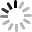# SVG 研究之路 (28) - filter - feDisplacementMap## feDisplacementMap

feDisplacementMap 的屬性：

• scale
• xChannelSelector
• yChannelSelector
• in
• in2

## 效果實作

``````<svg width="250" height="150">
<defs>
<filter id="f" filterUnits="objectBoundingBox" primitiveUnits="objectBoundingBox" x="0" y="0" width="1" height="1">
<feImage result="pict1" xlink:href="#m1" x="0" y="0" width="1" height="1"></feImage>
<feImage result="pict2" xlink:href="#m2" x="0" y="0"  width="1" height="1"></feImage>
<feDisplacementMap id="fdm" scale=".5" xChannelSelector="R" yChannelSelector="R" in2="pict2" in="pict1"></feDisplacementMap>
</filter>
<linearGradient id="g" x1="0" y1="0" x2="1" y2="1">
<stop offset="0" stop-color="#f00"></stop>
<stop offset="1" stop-color="#000"></stop>
<rect id="m2" x="0" y="0" width="100" height="100" fill="url(#g)"></rect>
<image id="m1" x="0" y="0" width="100" height="100" xlink:href="http://www.oxxostudio.tw/img/articles/201410/20141009_1_demo.jpg"></image>
</defs>
<rect x="0" y="0" width="100" height="100" filter="url(#f)" stroke="#000" transform="translate(110)"></rect>
<rect x="0" y="0" width="100" height="100" fill="url(#g)"></rect>
<rect x="110" y="0" width="100" height="100" fill="none" stroke="#000"></rect>
</svg>
````````````    <linearGradient id="g" x1="0" y1="0" x2="1" y2="1">
<stop offset="0" stop-color="#f00"></stop>
<stop offset="1" stop-color="#0f0"></stop>
````````````<feDisplacementMap id="fdm" scale=".5" xChannelSelector="A" yChannelSelector="A" in2="pict2" in="pict1"></feDisplacementMap>
````````````<feDisplacementMap id="fdm" scale=".5" xChannelSelector="B" yChannelSelector="B" in2="pict2" in="pict1"></feDisplacementMap>
````````````    <linearGradient id="g" x1="0" y1="0" x2="1" y2="1">
<stop offset="0" stop-color="rgba(255,0,0,0)"></stop>
<stop offset="1" stop-color="rgba(0,255,0,1)"></stop>
````````````    <image id="m1" x="0" y="0" width="100" height="100" xlink:href="http://www.oxxostudio.tw/img/articles/201410/20141009_1_demo.jpg"></image>
<image id="m3" x="0" y="0" width="100" height="100" xlink:href="http://www.oxxostudio.tw/img/articles/201410/20141009_1_demo2.jpg"></image>
````````````<svg width="340" height="231">
<defs>
<filter id="f" filterUnits="objectBoundingBox" primitiveUnits="objectBoundingBox" x="0" y="0" width="1.1" height="1.1">
<feImage result="pict1" xlink:href="#m1" x="0" y="0" width="1.1" height="1.1"></feImage>
<feImage result="pict2" xlink:href="#m2" x="0" y="0" width="1.1" height="1.1"></feImage>
<feDisplacementMap id="fdm" scale=".1" xChannelSelector="R" yChannelSelector="R" in2="pict2" in="pict1"></feDisplacementMap>
</filter>
<stop offset="0" stop-color="#000"></stop>
<stop offset="1" stop-color="#fff"></stop>
<rect id="m2" x="-10" y="0" width="410" height="300" fill="url(#g)"></rect>
<image id="m1" x="0" y="0" width="400" height="300" xlink:href="http://www.oxxostudio.tw/img/articles/201410/20141009_1_demo3.JPG"></image>
</defs>
<rect x="0" y="0" width="400" height="300" filter="url(#f)" transform="translate(-60 -60)"></rect>
</svg>
````````````<svg width="340" height="231">
<defs>
<filter id="f" filterUnits="objectBoundingBox" primitiveUnits="objectBoundingBox" x="0" y="0" width="1.1" height="1.1">
<feImage result="pict1" xlink:href="#m1" x="0" y="0" width="1.1" height="1.1"></feImage>
<feImage result="pict2" xlink:href="#m2" x="0" y="0" width="1.1" height="1.1"></feImage>
<feDisplacementMap id="fdm" scale=".05" xChannelSelector="A" yChannelSelector="A" in2="pict2" in="pict1"></feDisplacementMap>
</filter>
<stop offset="0" stop-color="rgba(0,0,0,.8)"></stop>
<stop offset=".2" stop-color="rgba(0,0,0,.7)"></stop>
<stop offset=".6" stop-color="rgba(0,0,0,.1)"></stop>
<stop offset="1" stop-color="rgba(0,0,0,0)"></stop>
<stop offset="0" stop-color="rgba(0,0,0,.8)"></stop>
<stop offset=".2" stop-color="rgba(0,0,0,.7)"></stop>
<stop offset=".6" stop-color="rgba(0,0,0,.1)"></stop>
<stop offset="1" stop-color="rgba(0,0,0,0)"></stop>